# Nuclear Chemistry Assignment Help

## What is nuclear chemistry?

Chemistry is a mix of many phenomena, some chemical and some physical. we all have heard of the attacks by US planes on docks of Japan with nuclear bombs named “Fat Man” and “Little Boy”. The level of destruction and remorse had been painful. 30 years later the same Japan used that destructive element for generation of electricity. So, this is how chemistry is, a small change in concentration could lead to a huge change in output. The topic “Nuclear Chemistry” revolves around changes with the nucleus of an element. Nuclear chemistry is the study of change in the nucleus of an element when the nucleon is disturbed and removal of either protons or neutrons result in huge energy release. Being biologically hazardous their usage for destructions had then stopped, yet countries rely on nuclear weapons as their last hopes.

## How nuclear chemistry came to spotlight?

Many experiments were conducted, scientists like Henri Becquerel and Wilham RÖNTGEN dedicated their majority of life searching for a magical element, that would release energy on its own. Madam Marie Curie and her husband Pierre Curie had discovered two new elements out of uranium ore, using radiometric methods. The two elements obtained by the Curie pair were:

(a) Polonium(Po)[atomic number=84]

Experiments of English scientist Ernest Rutherford on gold foil had proven the existence of nucleus in the center of an atom. The radioactive decay followed first order kinetics and hence only had a half-life not a completion of the reaction. This way all radioactive reactions were first order kinetic reactions.

## Nuclear Chemistry Assignment Help By Online Tutoring and Guided Sessions from AssignmentHelp.Net

And that’s how radioactivity, as well as nuclear chemistry, came into existence.

### Key Topics under Nuclear chemistry:

Nuclear chemistry includes the study of the radioactivity as well as the radioactive decay and release of energy. The topics are:

1. Binding energy and relation with modern physics.
2. Kinetics of a radioactive process.
3. Nuclear equations.
4. Types of decay:
1. (a)Alpha decay
2. (b)Beta decay
3. (c)Gamma decay
5. stability of the nucleus.
6. nuclear transmutation.
7. dating.
8. Uses

### IN BRIEF:

Reactions involved in nuclear chemistry are radioactive in nature, that is; they radiate energy in the form of heat or light. Processes associated with such reaction are “nuclear fusion” and “nuclear fission”.

Nuclear fusion: Type of reaction in which two or more nuclei come close enough to form a new type of atomic nuclei. The heat liberated is due to the difference in mass of products and reactants.

For example Deuterium(D) and Tritium(T) neutron fuse to form a Helium nucleus.

*NOTE: Deuterium and Tritium are isotopes of Hydrogen; also known to be heavy Hydrogen.

Nuclear fission: Type of reaction in which one nucleus generates two or more daughter nuclei. This involves splitting of a neutron into lighter. As there is the release of free neutrons and gamma photons, a large amount of energy is released.

For example Uranium -235 introduced with a neutron would result in nucleus splitting which on further proceeding releases energy. This reaction continues for long orders. Such reactions are termed as “chain reactions”. These reactions follow 1st order kinetics and hence their completion period is not known.

*NOTE: Chain Reaction- A chain reaction occurs when one single nuclear reaction causes an average of one or more subsequent nuclear reactions, thus leading to the possibility of a self-propagating series of these reactions.

As from above read concepts, it is clear that nuclear chemistry deals with the release of energy when, either a radioactive element combines with a neutron or it splits into daughter nuclei.

### 1. Binding energy:

As we know that our nature is so grateful to us all the times and hence believes in equality and balance, on the basis of which “law of mass and energy conservation” was introduced. During a radioactive decay, the energies released were due to the differences in mass of reactant and product, which varied from the original masses of the compounds, thus the concept of “MASS DEFECT” was introduced.

Mass defect: It is defined as the difference between the mass of an isotope and its mass number.

Binding energy: It is the energy that holds on a nucleus together which is equal to the mass defect of the nucleus.

To understand this concept, some help of the modern physics would be asked.

Would have heard of “energy-mass equivalence”, the famous Einstein’s equation which revolutionized the concepts of science and technology.

E = mc^2
*m= mass of the substance.
c= speed of light (3×10^8 m/s).
E= Energy released/absorbed by the substance.

To calculate the binding energy associated with a radioactive decay Einstein’s equation of energy-mass equivalence is applied. Here equation is accordingly modified and the relation between binding energy and the mass defect is expressed.

E = ∆mc^2
∆m= mass defect.
E= binding energy.

Graphical representation of binding energy with a mass number of various elements is shown ahead: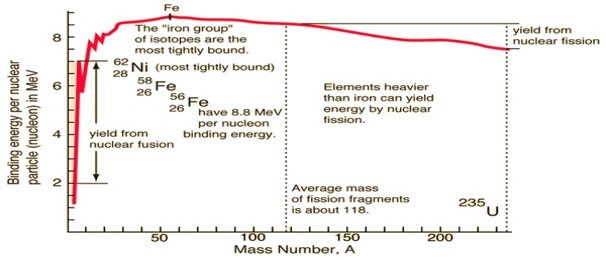The graph shows that as the mass number increases till Fe(Z=26) binding energy curve rises and then remains constant till radioactive substances.

### Sample Problem:

Question: Calculate the binding energy of U (235) if the mass of this nuclide is 235.0349 amu.

Solution

A neutral U (235) atom contains 92 protons, 92 electrons, and 143 neutrons. The predicted mass of a U (235) atom is therefore 236.9601 amu, to four significant figures:

[92(1.00728) amu] + [92(0.0005486) amu] + [143(1.00867) amu] = 236.9601 amu
total mass = 236.9601 amu
To calculate the mass defect for this nuclide, we subtract the observed mass from the predicted mass:
236.9601 amu - 235.0349 amu = 1.9252 amu
mass defect = 1.9252 amu
Using the conversion factor that relates the binding energy to the mass defect, we obtain a binding energy for 235U of 1793.3 MeV per atom:
1.9252 amu /1 atom = 931.50 MeV/1amu 1793.3 MeV/atom

### Kinetics of a Radioactive Process:

Radioactive substances follow chain reaction mechanism that continues for very long orders. Hence their complete life cycle could not be determined, the half-life for the same could be determined on the basis of which it was concluded that radioactive activities follow first order kinetics only.

In a first order reaction, the rate depends on the change in concentration of only one reactant. The rate law equation for a first order equation is:

R=k[A]
R= rate of reaction.
k= reaction rate constant
[A]= concentration of reactant.

As the radioactive decay proceeds the reactant concentration decreases and so does the reaction rate.

For a radioactive decay using the integrated rate law equation, the half-life and 99% completion of the reaction could be computed. Formulae used are as follows:

k = (2.303/t)log[a/a-x]
Where t= time at which reaction rate is being determined.
a= initial concentration of the reactant
x= concentration of reactant at time=t.

using this equation, the rate of reaction, half-life and 99% completion of reaction could be computed.

For half-life, the formula would be k = (0.693)/(t(half))
For 99% completion, the formula would be k=(2.303)/t
Here t = time taken for 99% completion of the reaction.
Hence the above-studied points explain the kinetics of a radioactive decay.

### Nuclear equations:

Similar to the chemical equations a nuclear equation includes symbols of elements, arrows to show the direction of reaction plus it also includes the description of the mass number and an atomic number of the radioactive element.

The principle difference between a chemical and nuclear reaction lies in how the reaction occurs, specifically how the atom is affected. Chemical reactions involve an atom’s electrons while nuclear reactions involve the atom’s nucleus.

#### Writing a nuclear equation:

In order to write an equation for a nuclear reaction, we must first establish some basic rules. Each of the elements involved in the reaction is identified by the chemical symbol. Two numbers are attached to the symbol. The number at the upper right is the mass number(A). The {'A'} number describes the atomic weight of the atom and identifies the number of protons and neutrons in the nucleus. The number at the lower left is the atomic number(Z). The {'Z'} number describes the number of protons in the nucleus and determines the type of atom.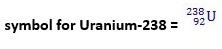This shows you that Uranium has a mass number of 238 and an atomic number of 92.

Symbols are also utilized to represent alpha and beta particles.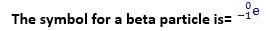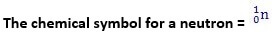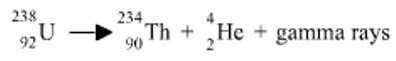Writing a nuclear equation needs knowledge of symbols, mass numbers and atomic numbers, which has now been provided.

Types of decay: as we observed that in a radioactive decay there is the release of high volume energy along a with-it generation of new nuclei and nucleons. Each decay lies in the category of one of the following three types of decay:

1. Alpha decay
2. Beta decay
3. Gamma decay

They have been named so on the basis of the type of particle decayed during the fission process.

Alpha decay: During the alpha decay, an alpha particle is dissociated whose specifications are:

Composition: 2 protons, 2 neutrons
Classification: Boson
Symbol: α, He²⁺, α²⁺
Mass: 4.001506179125(62) u, 3.727379240(82) GeV/c², 6.64465675(29)×10⁻²⁷ kg
Electric charge: 2 e
Spin: 0
These characteristics are similar to those of a HELIUM(He) particle.

Beta Decay: beta decay (β-decay) is a type of radioactive decay in which a beta ray (fast energetic electron or positron), and a neutrino are emitted from an atomic nucleus. For example, Potassium(K)-40. Beta decay is a consequence of the weak force, which is characterized by relatively lengthy decay times. Beta decay is of two types:

(a) β(+): in beta plus decay, a proton is converted to a neutron and the process creates a positron and an electron neutrino. Example as shown below: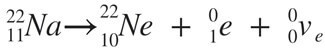(b) β(-): In beta minus decay, a neutron is converted to a proton and the process creates an electron and an electron antineutrino. Example as shown below: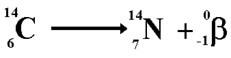Gamma Decay: An excited nucleus decays by the emission of α or β particle. The daughter nucleus that results is usually left in an excited state. It can then decay to a lower energy state by emitting a gamma ray photon. This process is called gamma decay. Examples as shown below: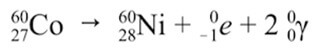### Stability Of nucleus:

There are no concrete theories to explain this, but there are only general observations based on the available stable isotopes. It appears that neutron to proton (n/p) ratio is the dominant factor in nuclear stability. This ratio is close to 1 for atoms of elements with low atomic number and increases as the atomic number increases. Then how do we predict the nuclear stability? One of the simplest ways of predicting the nuclear stability is based on whether nucleus contains odd/even number of protons and neutrons:

ProtonsNeutronsNumber of stable nuclides
OddEven04
OddOdd50
EvenEven57
evenOdd168

This shows that stability increased moving down the table.

Nuclear transmutation: ever thought of buying gold, remember that gold can be turned to lead, with the help of some basic chemicals and requisite knowledge. So, the process of turning an isotope of any element into another by application of nuclear reactions is termed as nuclear transmutation. As all elements have their atomic number as their identity, which is equal to the number of protons present in the nucleus, hence to conduct nucleus-transmutation, the number of protons in the nucleus is to be changed. The process needs the involvement of higher energy grades, that could be achieved only by energy released by nuclear reactions.

Example: It transpired that, under true nuclear transmutation, it is far easier to turn gold into lead than the reverse reaction, which was the one the alchemists had ardently pursued. Nuclear experiments have successfully transmuted lead into gold, but the expense far exceeds any gain.

Dating: how do we guess the age of a fossil found beneath the crust which had been lying there for years that is unknown, yet their age is determined to apply the concepts of “carbon-dating”.

Carbon dating: the determination of the age or date of organic matter from the relative proportions of the carbon isotopes carbon-12 and carbon-14 that it contains. The ratio between them changes as radioactive carbon-14 decays and is not replaced by exchange with the atmosphere.

Methods earlier applied: the first method for dating organic objects (such as the remains of plants and animals) was developed by another American chemist, Willard Libby (1908–1980). He became intrigued by carbon–14, a radioactive isotope of carbon. Carbon has isotopes with atomic weights between 9 and 15. The most abundant isotope in nature is carbon–12, followed in abundance by carbon–13. Together carbon–12 and carbon–13 make up 99% of all naturally occurring carbon. Among the less abundant isotopes is carbon–14, which is produced in small quantities in the earth's atmosphere through interactions involving cosmic rays. In any living organism, the relative concentration of carbon–14 is the same as it is in the atmosphere because of the interchange of this isotope between the organism and the air. This carbon–14 cycles through an organism while it is alive, but once it dies, the organism accumulates no additional carbon–14. Whatever carbon–14 was present at the time of the organism's death begins to decay to nitrogen–14 by emitting radiation in a process known as beta decay. The difference between the concentration of carbon–14 in the material to be dated and the concentration in the atmosphere provides a basis for estimating the age of a specimen, given that the rate of decay of carbon–14 is well known. The length of time required for one-half of the unstable carbon–14 nuclei to decay (i.e., the half-life) is 5,730 years.

These methods helped in determining the age of a fossil and other organic compounds just by determining the ratio of carbon and their isotopes.

After reading these all concepts and facts, a clear image of nuclear chemistry would be generated. Application of chemical kinetics, modern physics, and Einstein’s equation helped in clearing the obstacles of binding energy and energy barriers. This branch of chemistry has both productive as well as destructive outcomes, depends on the reader and how does one interpret this knowledge.

Some general uses of the Nuclear Chemistry are listed below, please go through them and get the practical approach toward this subject.

### Uses of radioactive decay and nuclear chemistry:

Cancer Treatment: Alpha particle radiations are used to attack at the infected site, this therapy is known as Radiotherapy, and element used is Radium-226. During the surgery, only the infected site is attacked and the remaining healthy cells go unaffected. Alternative radiations have also been applied using elements like Cobalt-60.

Static Eliminator: Radiations from Polonium-210 are applied to eliminate static local electricity in industrial and commercial industries. The positive charges from alpha radiation attract the negatively charged electrons, thereby reducing the potential of local static electricity.

Smoke Detector: Alpha particles from Americium-241 are bombarded with air molecules thereby releasing electrons. The free electrons generate a current flow. Smoke disrupts the current flow and fire alarm is blown.

Spacecraft power: Spacecraft and satellites have thermoelectric generators which have a similar working principle as solar panels. This device functions like a battery, with the benefit of a longer life span. Plutonium-238 serves as the fuel source, producing alpha radiation resulting in heat, which is converted to electricity.

Pacemaker Battery: Alpha radiation is used as an energy source to power heart pacemakers. Plutonium-238 is used as the fuel source for such batteries. This source of power provides a long lifespan for pacemakers. Being toxic in nature, these batteries have now been discarded as they had after effects that affected the health of the patient.

Remote sensing stations: Different military groups in various countries use alpha radiation to power remote sensing stations in far-off areas. Strontium-90 is typically used as the fuel source. These alpha radiated systems reduce the human effort and risk of life in such areas with unmanned surveillance, that too for longer periods of time.

Heating devices: Radiations are applied in various industries where a larger amount of heat is required in the shorter period of time. Such radiations are hard to control and are generally used in nuclear power plants and electricity generation plants.

Oil Well Equipment: Oil industries have to make oil rigs and refineries in remote areas where the source of power for generators and equipment could not be obtained. Hence in such areas, radiations and nuclear discharges are used as power source to run the plant. Strontium-90 is the typical fuel source in this sector.